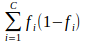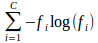# Matching with machine learning from an algorithmic standpoint - 7.2

author
Talend Documentation Team
EnrichVersion
Cloud
7.2
EnrichProdName
Talend Big Data Platform
Talend Data Fabric
Talend Real-Time Big Data Platform
Data Governance > Third-party systems > Data Quality components > Matching components > Matching with machine learning components
Data Quality and Preparation > Third-party systems > Data Quality components > Matching components > Matching with machine learning components
Design and Development > Third-party systems > Data Quality components > Matching components > Matching with machine learning components
EnrichPlatform
Talend Studio

## Matching with machine learning from an algorithmic standpoint

Matching with machine learning involve using the following matching components:
• tMatchPairing
• tMatchModel
• tMatchPredict

To get an overview of the workflow used to predict matches for datasets automatically, see The machine learning approach.

## How does tMatchPairing compute the suspect duplicate pairs?

The tMatchPairing component uses the Suffix Array Blocking method, which can be seen as a fuzzy blocking method, to compute the suspect duplicate pairs

The main steps of the process are the following ones:

1. For each row, the values of the different columns used as a blocking key are concatenated. Then, all the suffixes of length equal or greater than the value set for the Min suffix length parameter are generated. By default, the value is set to 3 in Talend Studio.

For example, the first_name and last_name columns are used as a blocking key. The first_name column contains the value `John` and the last_name column contains the value `Doe`. Then, the suffixes generated are `Doe`, `nDoe`, `hnDoe`, `ohnDoe` and `JohnDoe`.

2. The remaining suffixes are sorted alphabetically. If two consecutive suffixes are too similar, they are merged.

If the number of rows having a given suffix exceeds the value set for the Max block size parameter, this suffix is considered to be too frequent. It is then removed.

3. For each suffix, all the possible pairs of rows with records having the same suffix are generated. The value set for the Max block size parameter should not be too large because the number of combinations can dramatically increase. By default, the value for the Max block size parameter is set to 10.

4. The last step is the filtering step. It consists of removing the pairs that are less likely to match. A score for each pair is computed and added in the output schema. To compute a score for each pair of suspect duplicates, a sample of fixed size is generated. The default size is set to 10000. The two following steps are applied to the sample:

• Computing different measures - Levenshtein, Jaro-Winkler and Exact without case - for each pair and each column.

• Computing the percentile for each pair of suspect duplicates and each column.

It is now possible to give a very good approximate value for the percentile, given a value for a measure. On the global dataset, the different measures are computed for each pair and the percentile is retrieved for each measure. Then the score is computed using two steps:

• Computing the maximum percentile over measures for each column

• Computing the minimum percentile over columns.

If the score is lower than the threshold, the pair is filtered. This guarantees that each column has at least one measure above the threshold, meaning that all the columns are matching with respect to at least one measure.

## How does tMatchPairing compute the sample of suspect duplicate pairs?

The list of suspect duplicate pairs can be very large. You label only a subset of this list to identify the potential groups of duplicates.

You can then use machine learning to predict labels for the whole list. Then, it is possible to output a sample of this list, with a size fixed manually. The sample is chosen randomly.

For an example of how to label suspect pairs in a Grouping campaign created in Talend Data Stewardship, see Handling grouping tasks to decide on relationship among pairs of records.

## Extracting matching features using tMatchModel

You can use the labeled sample of suspect duplicate pairs as the input of the tMatchModel component.

You have to specify the set of columns the model will be built on and the column specifying the label. The algorithm will compute different measures, called features, to catch as much information as possible on this set of columns.

The tMatchModel component uses the Random forest algorithm to build the model. This algorithm is a generalization of decision trees.

## Analyzing data using decision trees and the Random forest algorithm

Decision tree is a greedy algorithm that performs a recursive binary partitioning of the feature space.

Each partition is chosen greedily by selecting the best split from a set of possible splits, in order to maximize the information gain at a tree node. Information gain is linked with the node impurity, which measures the homogeneity of the labels at a given node.

The current implementation provides two impurity measures for classification:

• Gini impurity• Entropywhere fi is the frequency of label i at a node.

In order to split, each continuous feature has to be discretized. The number of bins of each feature is managed by the Max Bins parameter. Increasing the value for this parameter allows the algorithm to consider more split candidates and make fine-grained split decisions. However, it increases computation. The decision tree construction stops as soon as one of the following conditions is met:

• The node depth is equal to the maximum depth of a tree. Deeper decision trees are more expressive, but they are more resource consuming and are prone to overfitting.

• No split candidate leads to an information gain greater than Min Info Gain.

• No split candidate produces child nodes which each have at least Min Instance Per Node rows.

The Random forest algorithm runs the decision tree algorithm many times (controlled by parameter `numTrees`), over a subset of the dataset and a subset of the features.

The two subsets are handled by two parameters:

• Subsampling rate: This parameter specifies the fraction of the input dataset used for training each decision tree in the forest. The dataset is created by conducting sampling with replacement, meaning that a row can be present many times for example.

• Subset strategy: This parameter specifies the number of features to be considered for each tree. You can specify one of the following values:
• auto: the strategy is automatically based on the number of trees in the forest.

• all: the total number of features is considered for split.

• sqrt: the number of features to be considered is the square root of the total number of features.

• log2: the number of features to be considered is the result of log2(M), where M is the total number of features.

This will generate different trees. New values can be predicted by taking the majority vote over the set of trees.

## Testing the model using the K-fold cross-validation technique

The K-fold cross-validation technique consists of assessing how good the model will be on an independent dataset.

To test the model, the dataset is split into `k` subsets and the Random forest algorithm is ran `k` times:

• At each iteration, one of the `k` subsets is retained as the validation set and the remaining `k-1` subsets are the training set.

• A score for each of the `k` runs is computed and then the scores obtained are averaged to calculate a global score.

## Tuning the Random forest algorithm hyper-parameters using grid search

You can specify values for the two Random forest algorithm hyper-parameters:

• The number of decision trees

• The maximum depth of a decision tree

To improve the quality of the model and tune the hyper-parameters, grid search builds models for each combination of the two Random forest algorithm hyper-parameter values within the limits you specified.

For example:

• The number of trees ranges from 5 to 50 with a step of 5; and

• the tree depth goes from 5 to 10 with a step of 1.

In this example, there will be 60 different combinations (10 × 6).

Only the best combination of the two hyper-parameters values used to train the best model is retained. This measure is reported by the K-fold cross-validation.

## How does tMatchPredict predict values on a dataset?

Once the learning model is built, the tMatchPredict component can predict values on a dataset using the model it receives from the tMatchModel component.

The input records can be either paired or unpaired:

• If the input records are paired, the tMatchPredict component can label suspect duplicates automatically.

• If the input records have not been paired, use the pairing model generated by the tMatchPairing component to compute the pairs of suspect duplicates.

Rather than returning pairs, the component can also return groups of records that are matching one another, by adding a clustering step in the algorithm. You can define the clustering classes, which are generally the label corresponding to a match.

The algorithm used for clustering computes connected components of the graph where each vertex is a record. There is an edge between two vertices if the pair of records has the right label.

For example, if record A matches record B and record B matches record C, a group including records A, B and C is created even if record A and record C do not match.